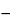Cholecalciferol Solution
DEFINITION
Cholecalciferol Solution is a solution of Cholecalciferol in an edible vegetable oil, in Polysorbate 80, or in Propylene Glycol. It contains NLT 90.0% and NMT 120.0% of the labeled amount of vitamin D as cholecalciferol (C27H44O).
ASSAY
•  Procedure
Mobile phase: Hexane and pentanol (997:3)
Standard stock solution: Dissolve USP Cholecalciferol RS in toluene, and dilute with Mobile phase to 50 µg/mL. [Note—Prepare this solution fresh daily. ]
Standard solution A: 5 µg/mL from Standard stock solution in Mobile phase. [Note—Store at a temperature not above 0. ]
Standard solution B: Transfer 5.0 mL of Standard stock solution to a round-bottom flask fitted with a reflux condenser. Displace the air with nitrogen, and reflux for 1 h in a water bath under a nitrogen atmosphere to obtain a solution containing cholecalciferol and precholecalciferol. Cool, transfer the solution with the aid of several portions of Mobile phase to a 50-mL volumetric flask, and dilute with Mobile phase to volume.
Sample solution: Equivalent to 5 µg/mL of cholecalciferol in Mobile phase from an accurately measured volume of Cholecalciferol Solution
Chromatographic system
Mode: LC
Detector: UV 254 nm
Column: 4.6-mm × 25-cm; packing L3
Flow rate: 2 mL/min
Injection size: 10 µL
System suitability
Sample: Standard solution B
[Note—The relative retention times for precholecalciferol and cholecalciferol are about 0.4 and 1.0, respectively. ]
Suitability requirements
Resolution: NLT 1.0 between the precholecalciferol peak and the cholecalciferol peak
Relative standard deviation: NMT 2.0%
Analysis
Samples: Standard solution A, Standard solution B, and Sample solution
Cholecalciferol response factor
Calculate the cholecalciferol response factor, FC:
FC = CS/rS
 CS = = concentration of USP Cholecalciferol RS in Standard solution A (µg/mL) rS = = peak area of cholecalciferol from Standard solution A
Pre-cholecalciferol response factor
Calculate the concentration, C¢S, in µg/mL, of cholecalciferol in Standard solution B:
C¢S = FC × r¢S
 FC = = response factor for cholecalciferol r¢S = = peak area of cholecalciferol from Standard solution B
Calculate the concentration, C¢pre, in µg/mL, of pre-cholecalciferol:
C¢pre = CSC¢S
 CS = = concentration of USP Cholecalciferol RS in Standard solution A (µg/mL) C¢S = = concentration of cholecalciferol in Standard solution B (µg/mL)
Calculate the response factor, Fpre, for pre-cholecalciferol:
Fpre = C¢pre/rp
 C¢pre = = concentration of pre-cholecalciferol (µg/mL) rp = = peak response of pre-cholecalciferol from Standard solution B
Content of vitamin D
Calculate the percentage of the labeled amount of vitamin D as cholecalciferol (C27H44O) in the portion of the Cholecalciferol Solution taken:
Result = {[(FC × rC) + (Fpre × rpre)]/CU} × 100
 FC = = response factor for cholecalciferol rC = = peak area of cholecalciferol from the Sample solution Fpre = = response factor for pre-cholecalciferol rpre = = peak area of pre-cholecalciferol from the Sample solution CU = = nominal concentration of cholecalciferol in the Sample solution (µg/mL)
Acceptance criteria: 90.0%–120.0%